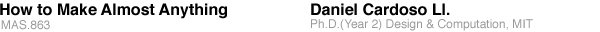sections contact email: dcardoso [at] sinmit [dot] edu

## The Melnikov Press-Fit Kit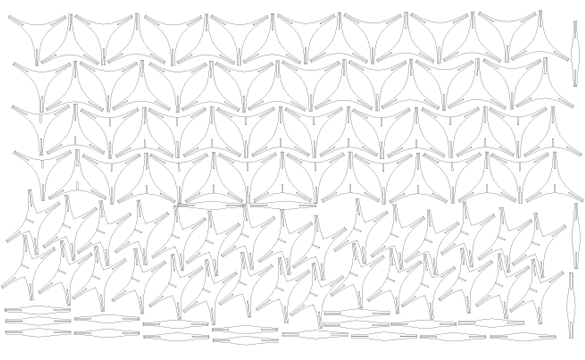Figure 1. The cutsheet for the Lasecutter looks like this.

This project is an exploration in 3-D of some simple geometric principles I am interested in since I started styudying Konstantin Melnikov's work (check some references to my previous work on Melnikov Grammar here). Melnikov's geometric principles work on the plane. Can they be translated to space?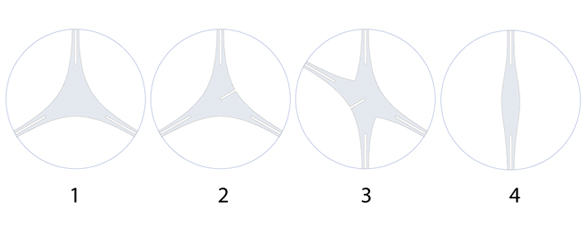Figure 2. The vocabulary of elements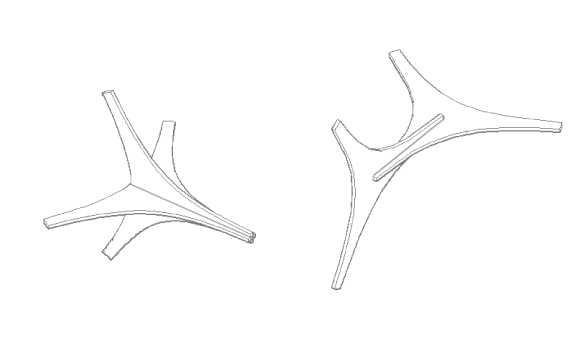Figure 3. The compound types

It was very difficult to foresee the kind of artifacts it would generate. I wanted to create an open system.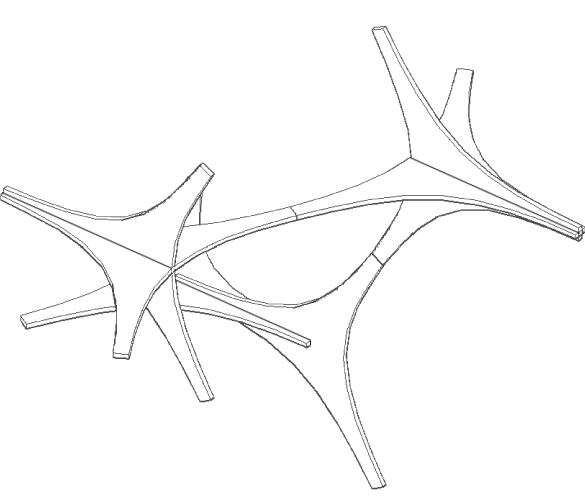Figure 3 and 4. Some simple derivations of the 3D 'Melnikov Grammar'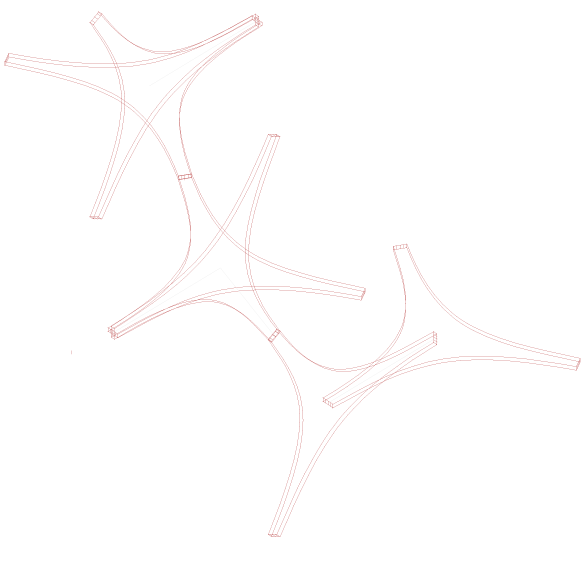Some photos of the first prototypes: I used 1/16 in chipboard, with a tolerance value of 0.007 in (at each side of the slot).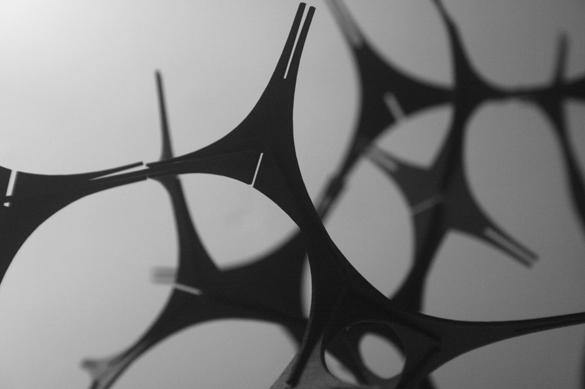Figure 5. An idea for the assembly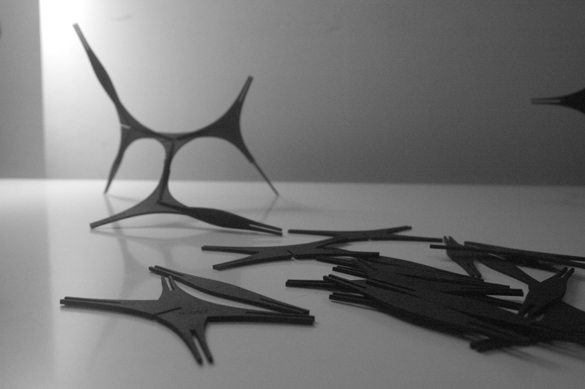Figure 6. Some pieces and a structure.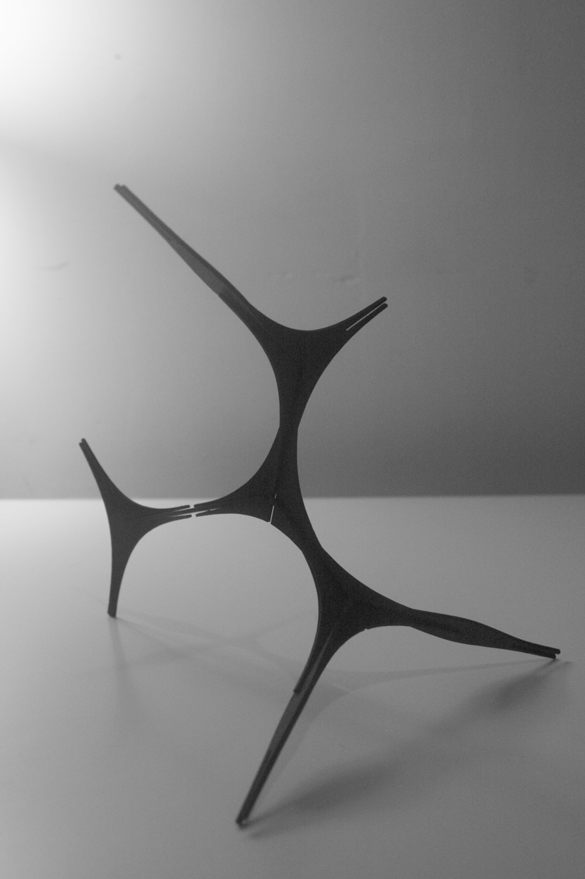Figure 7. Schematic section of the facade, detail and front view of a sensor’s node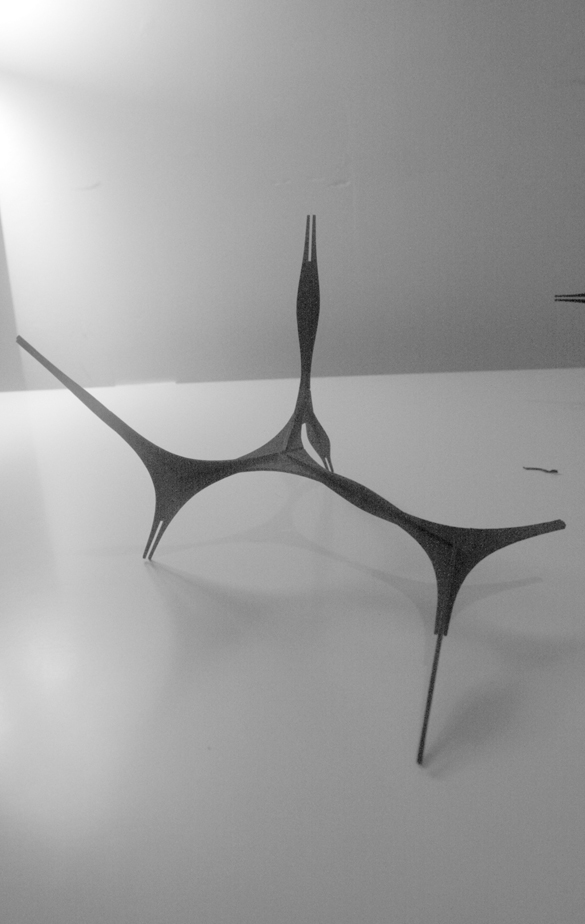Figure 8.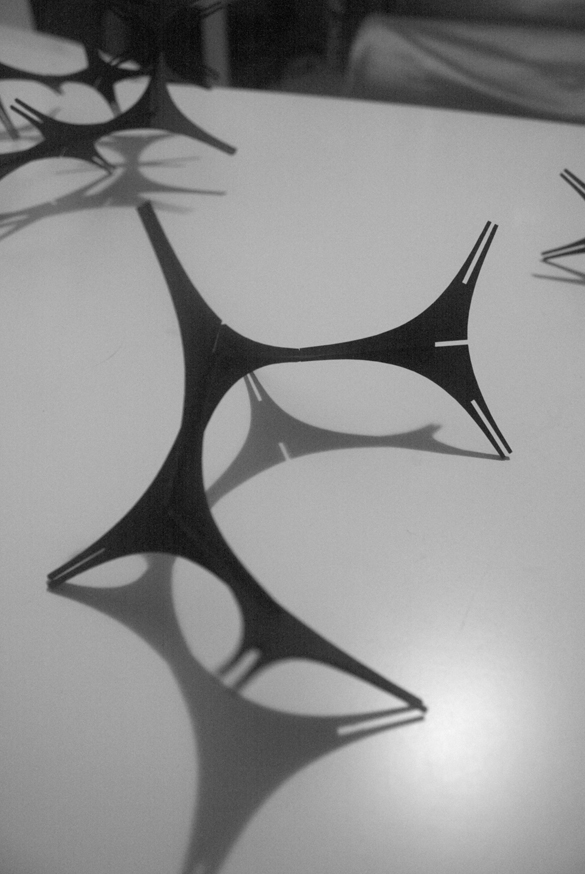Figure 9.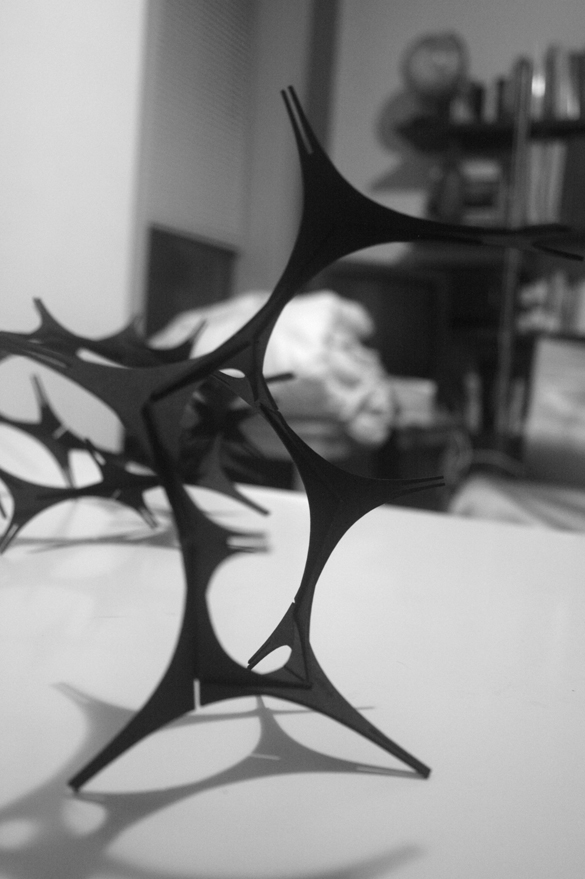Figure 10.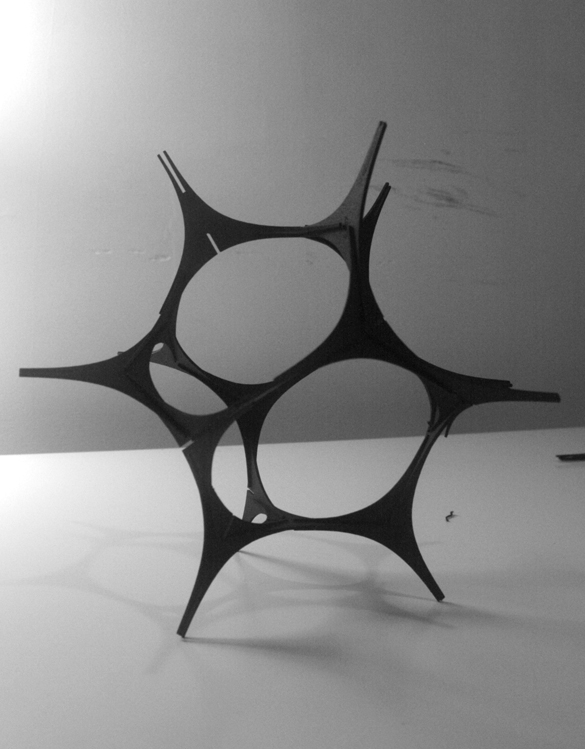Figure 11.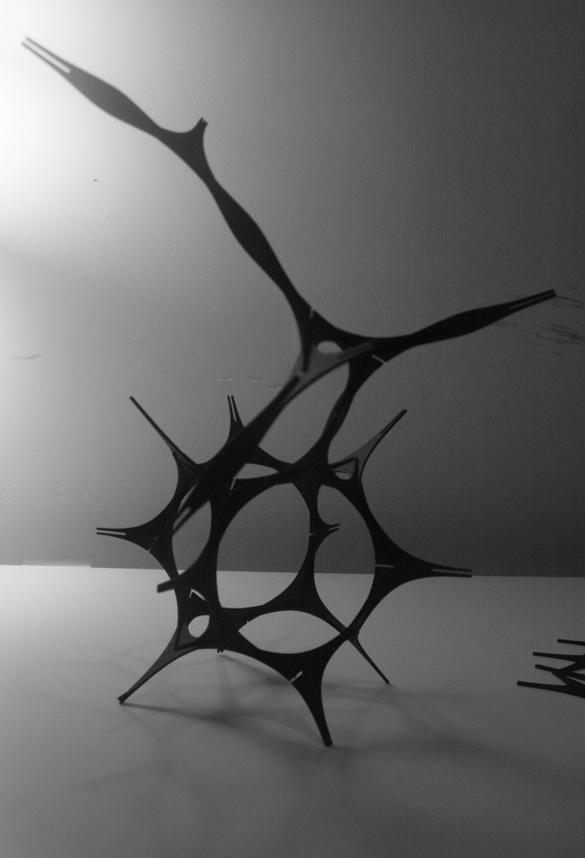Figure.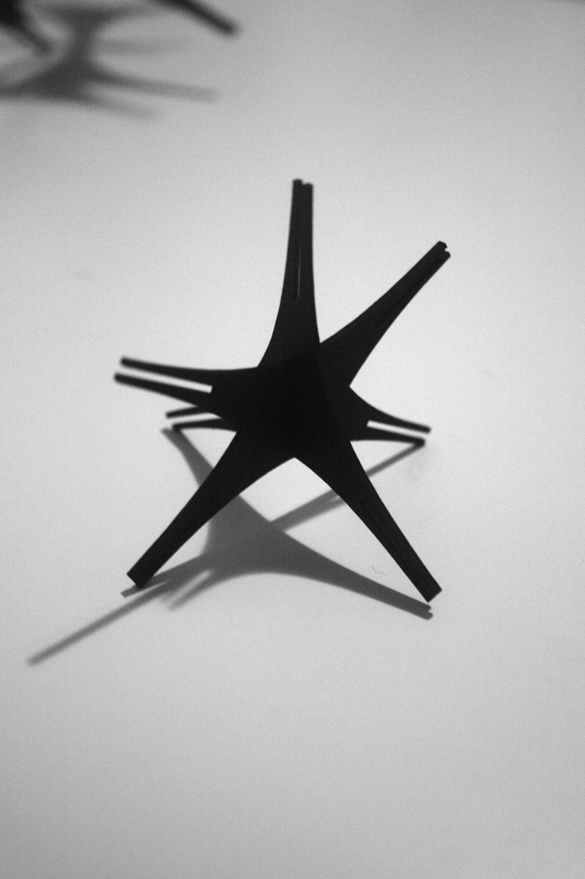Figure.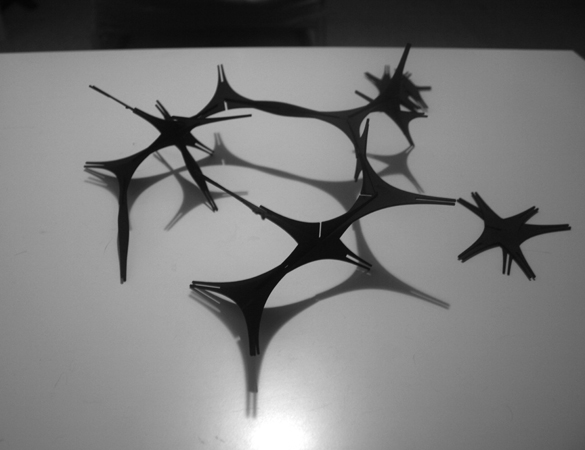Figure.Figure.

[ daniel cardoso site ]